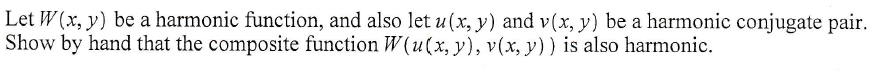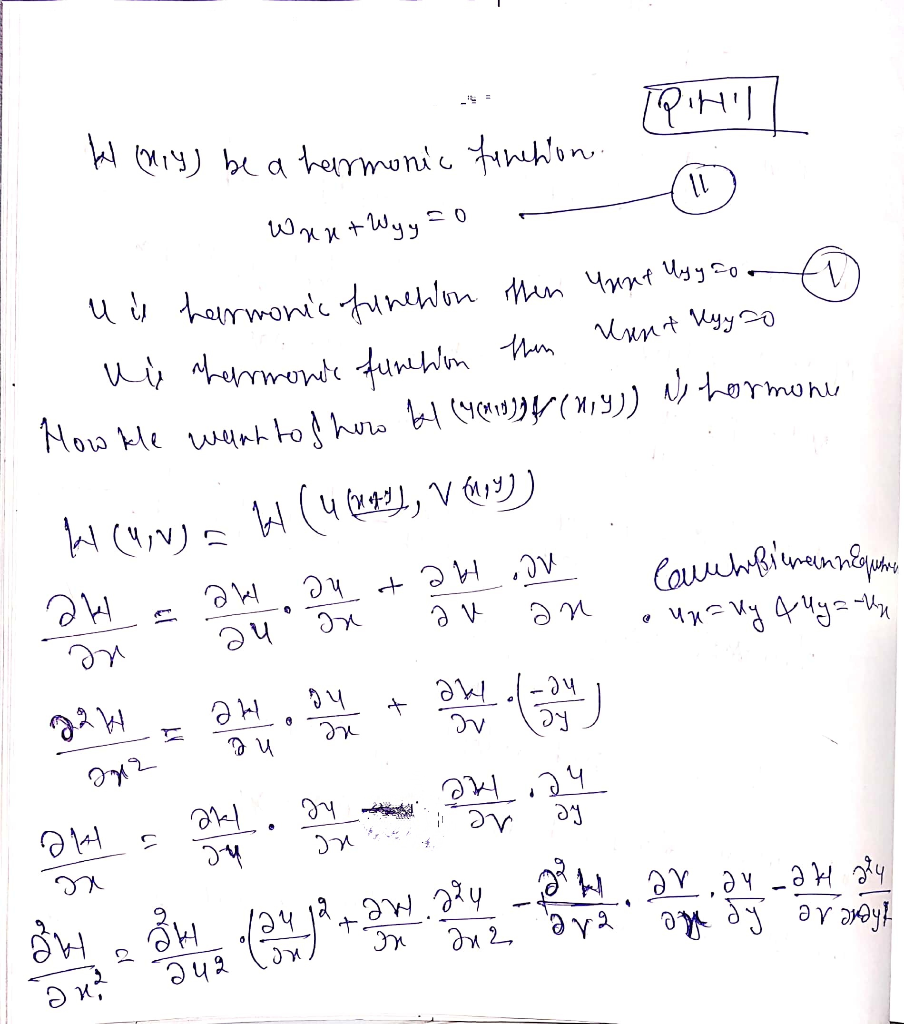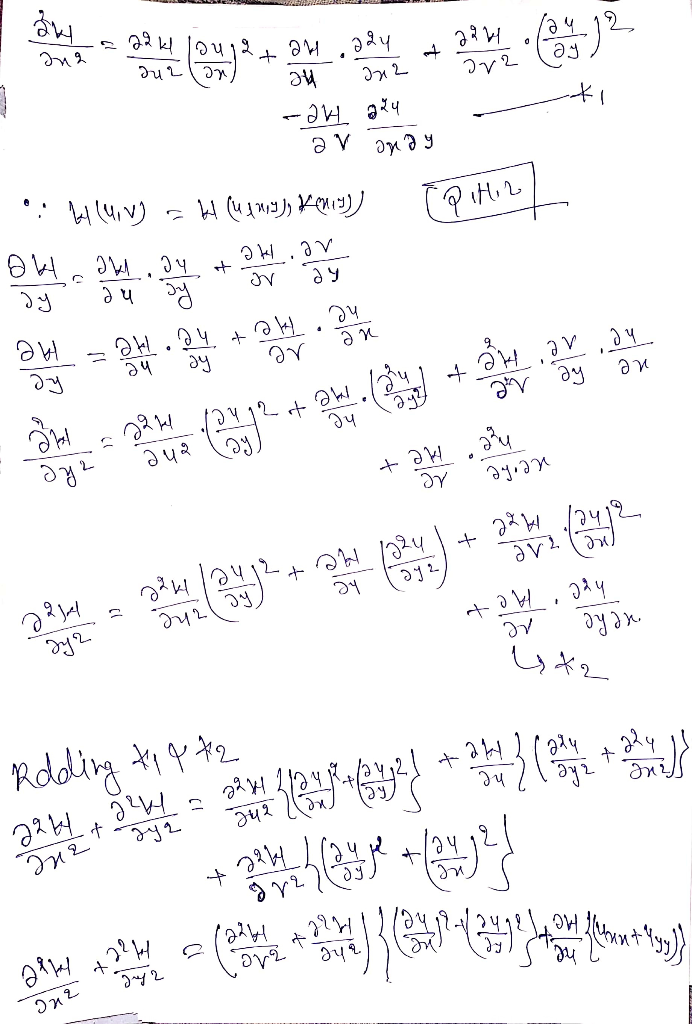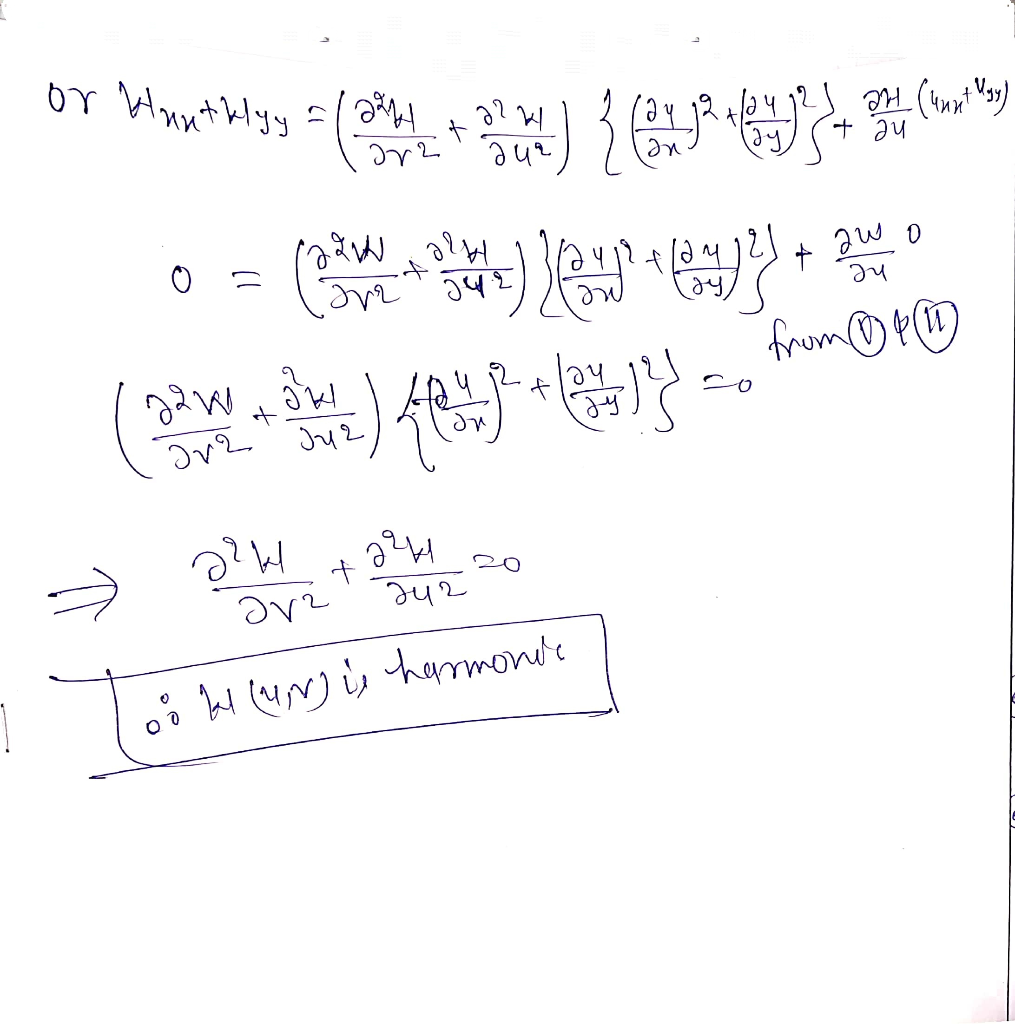Homework Help Question & Answers

# Let W(x, y) be a harmonic function, and also let u(x, y) and v(x, y) be a harmonic conjugate pair...Let W(x, y) be a harmonic function, and also let u(x, y) and v(x, y) be a harmonic conjugate pair. Show by hand that the composite function W(u(x, y), v(x, y)) is also harmonic.

#### Homework Answers

ReportAnswer #1Add Homework Help Answer
##### Add Answer of: Let W(x, y) be a harmonic function, and also let u(x, y) and v(x, y) be a harmonic conjugate pair...
Your Answer: Your Name: What's your source?
Not the answer you're looking for? Ask your own homework help question. Our experts will answer your question WITHIN MINUTES for Free.
More Homework Help Questions Additional questions in this topic.

• #### acids, conjugate acids, bases, conjugate bases.

Need Online Homework Help?

Get FREE EXPERT Answers
WITHIN MINUTES
Related Questions